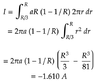# Electric Current and Resistance

• zoyash
In summary, there is a conversation about a problem involving current density across a cylindrical conductor and two different formulas are discussed: ##J=α(r−r^2/R)## and ##J=ar(1-\frac{1}{R})##. Both formulas seem to have dimensional errors and do not decrease linearly with r. The correct formula may be ##J=a(1-\frac{r}{R})##.

#### zoyash

Homework Statement
The current density across a cylindrical conductor of radius R varies according to the

equation J=α(r−r2/R), where r is the distance from the axis. Thus the current density is a

maximum J0 at the axis r=0 and decreases linearly to zero at the surface r=R in which α is a constant.

What is the current through the outer portion of the wire between radial distance R/3

and R. Take R=2mm and α=2*105 A/m2
Relevant Equations
J=α(r−r2/R)
I have attached my solution to this problem but I think I got the wrong answer.

#### Attachments

•math-20200507.png
8.6 KB · Views: 87
I read your post and the attached attempt at the solution but I have problem understanding which exactly is the formula for the current density. Can you write it in Latex? is it $$J(r)=\alpha r (1-\frac{r}{R})$$

Though in the solution it seems that you use as current density the formula below
$$J(r)=ar(1-\frac{1}{R})$$

I have to say that both formulas seem dimensionally incorrect since the units of ##\alpha## are given as A/m^2

Last edited:
•etotheipi
zoyash said:
Homework Statement:: The current density across a cylindrical conductor of radius R varies according to the

equation ## J=α(r−r^2/R) ## , where r is the distance from the axis. Thus the current density is a maximum J0 at the axis r=0 and decreases linearly to zero at the surface r=R in which α is a constant.
Your formula says J=0 at r=0. I think you may have meant ## J =J_0
\alpha (R - r^2/R) ##. Furthermore, in order for J to decrease linearly with r the formula would have to be something like ## J = J_0 \alpha(R-r). ## Try again.

Delta2 said:
in the solution it seems that you use as current density the formula below
$$J(r)=ar(1-\frac{1}{R})$$

I have to say that both formulas seem dimensionally incorrect since the units of ##\alpha## are given as A/m^2
Well, @zoyash started with $$J(r)=aR(1-\frac{1}{R})$$
But then switched to $$J(r)=ar(1-\frac{1}{R})$$
Neither makes sense dimensionally and neither decreases linearly with r. Probably should be $$J(r)=a(1-\frac{r}{R})$$

Last edited:
•etotheipi and Delta2